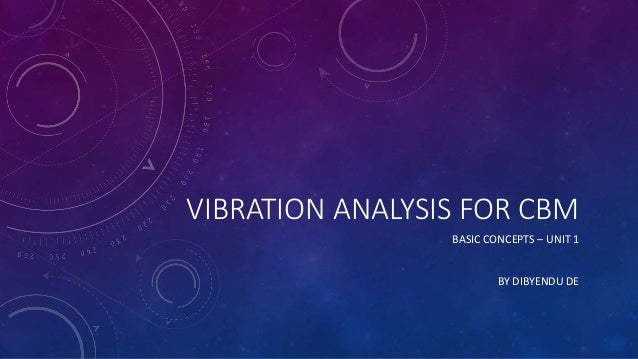Successfully reported this slideshow.
We use your LinkedIn profile and activity data to personalize ads and to show you more relevant ads. You can change your ad preferences anytime.Upcoming SlideShare
Loading in …5
×

# Vibration analysis unit1

This relates to a series of posts on Vibration Analysis. The purpose is education of engineers and managers to effectively improve availability, reliability and performance of their manufacturing plants and improve profitability of their business

• Full Name
Comment goes here.

Are you sure you want to Yes No
Your message goes here• Login to see the comments

### Vibration analysis unit1

1. 1. VIBRATION ANALYSIS FOR CBM BASIC CONCEPTS – UNIT 1 BY DIBYENDU DE
2. 2. ALL MACHINES VIBRATE Because of: Inertia Stiffness Imposed forces
3. 3. VIBRATION MONITORING What causes Vibration ? INDUCED FORCE & FREEDOM FOR MOVEMENT
4. 4. VIBRATION MONITORING Types of Forces ? Inertia Forces Acceleration & Deceleration Looseness & Impacts
5. 5. MACHINE VIBRATION IS COMPLEX • Behaviour of each component creates their own frequencies • Frequency tells us about a possible problem. • Amplitude tells us about the severity of the problem. • Multiple frequencies combine to produce the overall vibration • Vibration from 1 Hz to 1000 Hz captures overall amplitude
6. 6. ONE CYCLE OF VIBRATION • Movement of weight from neutral position to upper limit • Upper limit back through neutral position to lower limit • Lower limit to neutral position • The movement of the weight plotted against time is a sine wave •
7. 7. FREE & FORCED VIBRATION • With sudden impulse the system will vibrate at its natural frequency • Free vibrations die out with time if the system is stable. (Bump test) • Forced vibration are periodically imposed on the system (like motor). The response is also periodic
8. 8. FORCE, MOBILITY & VIBRATION • Response to forced vibration is not uniform at all frequencies. • Response depends on the mobility of the structure • Mobility varies with frequencies. High at resonance. Low where damping is present.
9. 9. MEASUREMENT OF AMPLITUDE & FREQUENCY Peak  for velocity and acceleration (used for algorithms) Peak to Peak  Displacement RMS  Velocity & acceleration (Europe) Energy content (only for sine wave) Average  Not used in vibration analysis Frequency = 1/Period (Hz/CPM)
10. 10. MEASUREMENT OF AMPLITUDE Peak  for velocity and acceleration Peak to Peak  Displacement RMS  Velocity & acceleration (Europe) Energy content (only for sine wave) Average  Not used in vibration analysis
11. 11. DISPLACEMENT, VELOCITY & ACCELERATION What? • Displacement = d = x = A sin (wt) • Velocity = v = dx / dt = Aw cos wt = Aw sin (wt + 90o) • Acceleration = a = dv /dt = - Aw2 sin wt = Aw2 sin (wt + 180o) Why? • Strain Energy ∝ to d2 • Kinetic Energy ∝ to v2 • Acoustic Pressure ∝ to a2 • Force
12. 12. VIBRATION - CAR ANALOGY Low Frequency Long period (T) High displacement Average Velocity Low Acceleration Medium Frequency Medium period (T) Average displacement Average Velocity Average Acceleration High Frequency Short period (T) Low displacement Average Velocity High Acceleration
13. 13. THANK YOU FOR COMPLETING UNIT 1 CONTACT: FOR FACILITATED TRAINING DIBYENDU DE, EMAIL ID: DDE337@GMAIL.COM